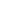Share

# Recursive Function

A recursive function is a function that calls itself during its execution. This enables the function to repeat itself several times, outputting the result and the end of each iteration. Below is an example of a recursive function.

function Count (integer N)
if (N <= 0) return "Must be a Positive Integer";
if (N > 9) return "Counting Completed";
else return Count (N+1);
end function

The function Count() above uses recursion to count from any number between 1 and 9, to the number 10. For example, Count(1) would return 2,3,4,5,6,7,8,9,10. Count(7) would return 8,9,10. The result could be used as a roundabout way to subtract the number from 10.

Recursive functions are common in computer science because they allow programmers to write efficient programs using a minimal amount of code. The downside is that they can cause infinite loops and other unexpected results if not written properly. For example, in the example above, the function is terminated if the number is 0 or less or greater than 9. If proper cases are not included in the function to stop the execution, the recursion will repeat forever, causing the program to crash, or worse yet, hang the entire computer system.

Published: 2006

Definition from the PC Glossary
https://pc.net/glossary/recursive_function